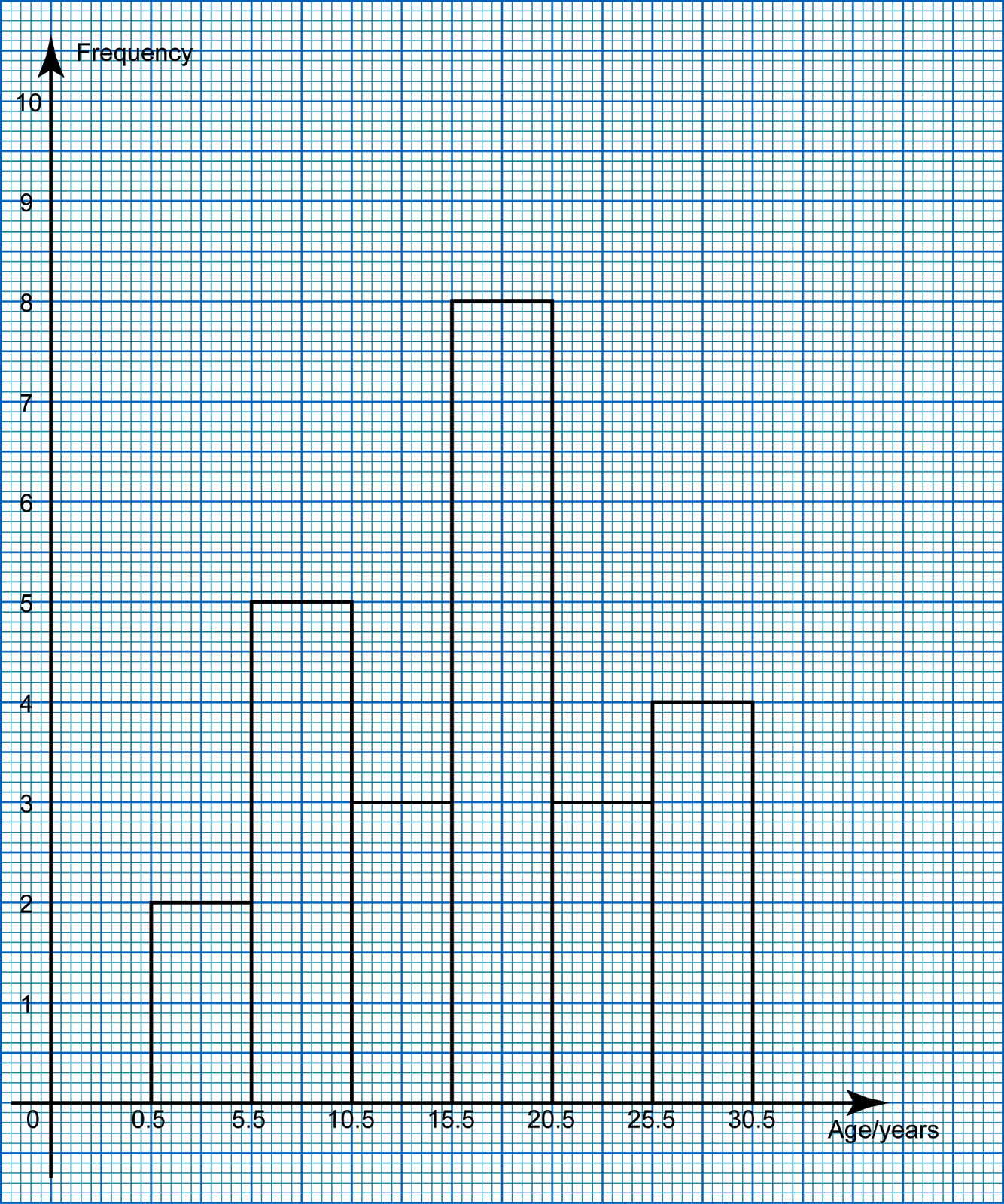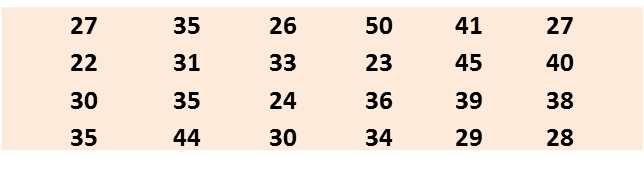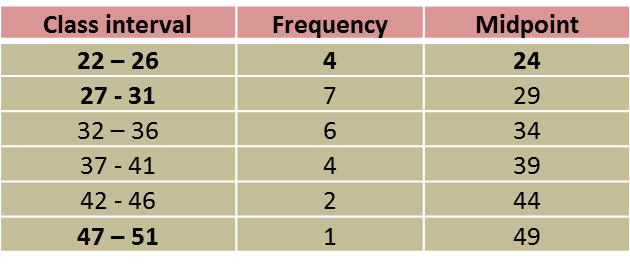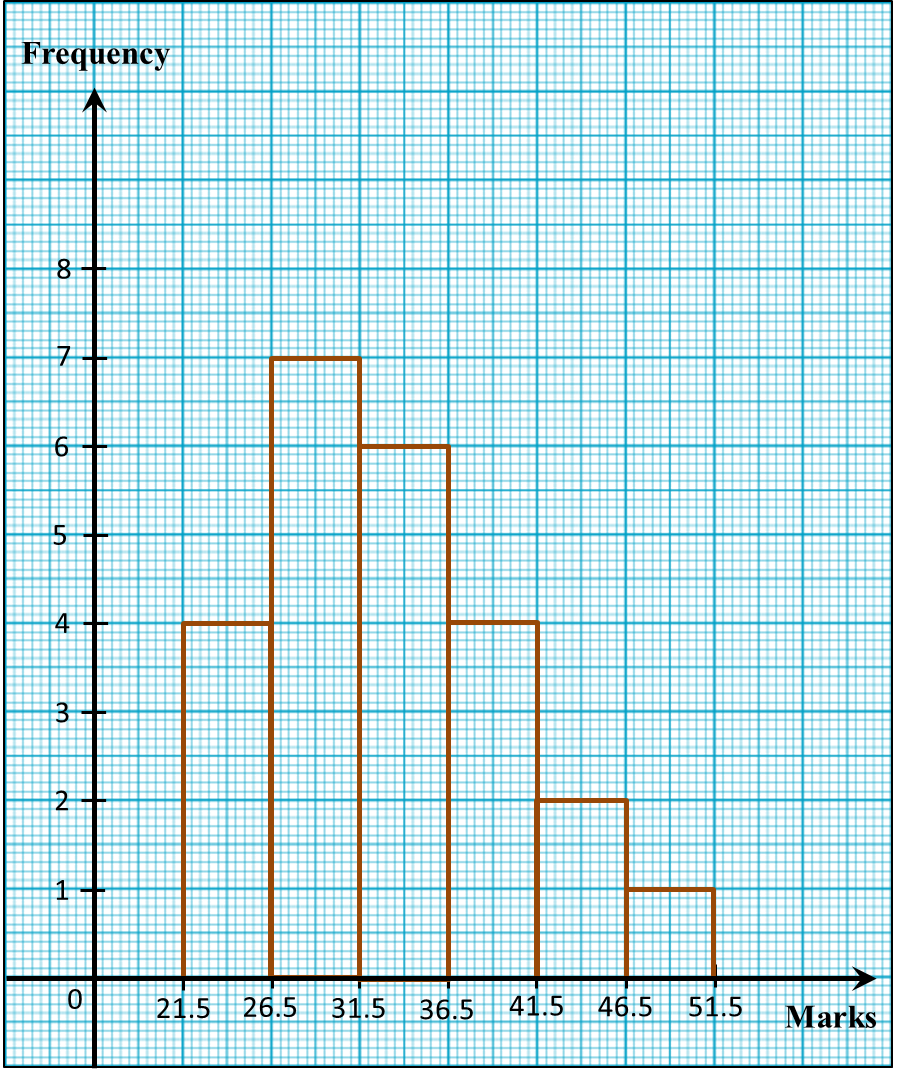# 7.4.1 Measures of Dispersion for Grouped Data, SPM Paper (Long Questions)

Question 1:
The data below shows the age of 25 tourists who visited a tourist spot.(a) Copy and complete the table below based on the data above.(b)
Based on the completed table above,
(i) State the modal class.
(ii) Calculate the mean age of the tourists.

(c) For this part of the question, use graph paper.
By using a scale of 2 cm to 5 years on the horizontal axis and 2 cm to 1 tourist on the vertical axis, draw a histogram for the data.

Solution
:

(a)Calculation of midpoint for (age 6 – 10) = $\frac{6+10}{2}=8$

(b)(i)
Modal class = age 16 – 20 (highest frequency)

(b)(ii)
$\begin{array}{l}\text{mean age =}\frac{3×2+8×5+13×3+18×8+23×3+28×4}{25}\\ \text{=}16.4\end{array}$

(c)Question 2:
Diagram below shows the marks, obtained by a group of 24 students in a mathematics quiz.(a) Based on the data in diagram above, complete Table in the answer space.

(b) State the modal class.

(c) Calculate the estimated marks obtained by a student.

(d) For this part of the question, use graph paper.
By using a scale of 2 cm to 5 marks on the horizontal axis and 2 cm to 1 student on the vertical axis, draw a histogram for the data.

(e) Based on the histogram drawn in (d), state the number of students who obtain less than 32 marks in the quiz.Solution
:
(a)(b) Modal class = 27 – 31 (highest frequency)

(d)(e)
Number of students who obtained less than 32 marks
= 4 + 7
= 11